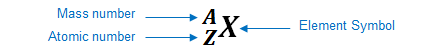# Problem: Chlorine has two naturally occurring isotopes, 35Cl and 37Cl. What is the mass number of each isotope? How many protons, neutrons, and electrons are present in each?

###### FREE Expert Solution

An element can be represented as:You can determine the identity of the element using its atomic number:

Atomic number (Z):

represents the identity of the element
reveals the number of protons
in a neutral element:

# of protons = # of electrons

Mass number (A)

reveals the number of protons and neutrons

mass number = # of protons + # of neutrons

86% (462 ratings)###### Problem Details

Chlorine has two naturally occurring isotopes, 35Cl and 37Cl. What is the mass number of each isotope? How many protons, neutrons, and electrons are present in each?

Frequently Asked Questions

What scientific concept do you need to know in order to solve this problem?

Our tutors have indicated that to solve this problem you will need to apply the Isotopes concept. You can view video lessons to learn Isotopes. Or if you need more Isotopes practice, you can also practice Isotopes practice problems.

What professor is this problem relevant for?

Based on our data, we think this problem is relevant for Professor Wagner's class at VT.

What textbook is this problem found in?

Our data indicates that this problem or a close variation was asked in Chemistry: The Molecular Nature of Matter and Change - Silberberg 8th Edition. You can also practice Chemistry: The Molecular Nature of Matter and Change - Silberberg 8th Edition practice problems.